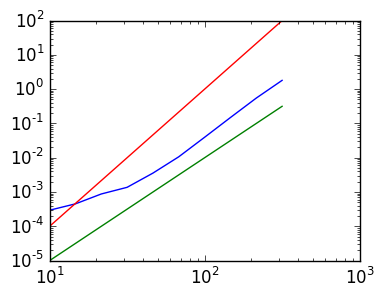# Lab 6: QR Decomposition using Householder reflections¶

In this lab, we construct an algorithm for calculating the QR Decomposition of a matrix $A$ using Householder reflections. In this lab we always use the 2-norm.

## Householder reflections¶

Given a vector $\mathbf v$ satisfying $\|\mathbf v\|=1$, the Householder reflection is the orthogonal matrix as

$$Q_{\mathbf v} \triangleq I - 2 \mathbf v \mathbf v^\top$$

Exercise 1(a) Demonstrate numerically that $Q_{\mathbf v}$ is orthogonal on a random vector of length 5 satisfying $\|\mathbf v\|=1$. Prove that this is always the case.

In :
v=rand(5)
v=v/norm(v)
Q=eye(5)-2v*v'

norm(Q*Q'-I)

Out:
6.364164569506414e-17

Exercise 1(b) Given n, how would you construct the length n basis vector $\mathbf e_1$? How about $\mathbf e_k$? (Advanced: do this exercise in one line using [v;w] syntax to concatinate two or more vectors, along with zeros to create vectors of zeros.) Show that your approach works for n=20 and k=5.

In :
n=20
k=5
e1=[1;zeros(n-1)]
ek=[zeros(k-1);1;zeros(n-k)];


Exercise 1(c) Given a vector $\mathbf x$, define

$$\mathbf w = \mathbf x - \alpha \|{\mathbf x}\| \mathbf e_1, \qquad \alpha = \pm 1,$$

where the choice of $\alpha$ is arbitrary. Demonstate numerically that

$$\|\mathbf w\|^2=2(\|x\|^2 - \alpha \|x \| x_1)$$

where $x_1 = \mathbf e_1^\top \mathbf x$, for a random vector $\mathbf x$ of length 5. (Advanced: Prove this is true.)

In :
n=5
x=rand(n)
α=1
w=x-α*norm(x)*[1;zeros(n-1)]

norm(w)^2-2(norm(x)^2-α*norm(x)*x)

Out:
2.220446049250313e-16

Exercise 1(c) With $\mathbf v = \mathbf w / \|\mathbf w\|$, demonstrate numerically that $Q_{\mathbf v}$ satsifies the property

$$Q_{\mathbf v}\mathbf x = \alpha \|{\mathbf x}\| \mathbf e_1.$$

In :
x=rand(10)
α=-1
w=x-α*norm(x)*[1;zeros(10-1)]
v=w/norm(w)
Q=I-2v*v'

Q*x - [1;zeros(9)]*norm(x)*α

Out:
10-element Array{Float64,1}:
-8.88178e-16
-1.83013e-16
-4.46691e-17
-1.71304e-16
-2.9924e-16
-6.72205e-17
-2.8276e-16
-1.78893e-17
-8.41341e-17
-5.55112e-17

## Calculating the QR Decomposition¶

Exercise 2(a) Use 1(c) to design a $\mathbf v$ so that $Q_{\mathbf v} A$ has zeros in the $2:n$ rows of the first column, where $A$ is an $n \times m$ matrix, where $n \geq m$. Demonstrate this work on a random $10 \times 5$ matrix.

In :
A=rand(10,5)

x=A[:,1]
α=-norm(x)
w=x-α*[1;zeros(10-1)]
v=w/norm(w)
Q=I-2v*v'

Q*A

Out:
10x5 Array{Float64,2}:
-1.95213      -1.67599    -1.42119    -1.2008     -1.56703
3.64292e-16  -0.0838621  -0.027715    0.268876   -0.169366
3.1225e-16    0.188906   -0.293262   -0.256776    0.0589008
1.72171e-16   0.343429   -0.139601    0.735957    0.522818
1.10589e-16  -0.165336    0.664247    0.291512    0.502147
2.35055e-16  -0.388994   -0.0455371   0.494435    0.222453
1.42247e-16   0.558234    0.523473   -0.0121894   0.652606
3.06179e-16  -0.253825    0.0771178   0.0125122  -0.204855
5.54244e-16  -0.426105   -0.276907   -0.109384    0.495433
1.11022e-16   0.022482    0.684233    0.775409    0.779559 

Exercise 2(b) Given $k<n$ and a vector $\mathbf x$ of length $n$, design a $\mathbf v$ so that

$$Q_{\mathbf v} \mathbf x = \begin{pmatrix} x_1 \cr \vdots \cr x_{k-1} \cr \alpha \|x[k:n]\| \cr 0 \cr \vdots \cr 0\end{pmatrix}.$$

Demonstrate that this works on a random vector of length 10 for k=3;

In :
k=3
n=10
x=rand(n)
α=-1
y=[zeros(k-1);x[k:end]]
v=y-α*norm(y)*[zeros(k-1);1;zeros(n-k)]
v=v/norm(v)
Q=I-2v*v'

norm(Q*x-[x[1:k-1];α*norm(x[k:end]);zeros(n-k)])

Out:
4.3071126364138936e-16

Exercise 2(c) How would you use 2(b) to construct to choose a $\mathbf v$ so that $Q_{\mathbf v} A$ has zeros in the $(j+1):n$ rows of the $j$th column, and but leaves the $1:(j-1)$ rows alone?

Exercise 2(d) How would you calculate the QR Decomposition using 2(c) with $m-1$ choices of $\mathbf v$ to, column-by-column, introduce zeros below the diagonal?

## Implementation¶

Exercise 3(a) Complete the following functions that returns a Householder reflection corresponding to exercise 2(c). Use α=-sign(x[j]). (This choice will be investigated next lab.)

In :
function house_col(A,j)
# TODO: Return a Householder reflection Q so that
#    QA=Q*A satsifies QA[j+1:n,j]==zeros(n-j) and QA[1:j-1,j]==A[1:j-1,j]
# for     n=size(A,1)
n=size(A,1)
x=[zeros(j-1);A[j:end,j]]
α=-sign(x[j])
v=x-α*norm(x)*[zeros(j-1);1;zeros(n-j)]
v=v/norm(v)
I-2v*v'
end

Out:
house_col (generic function with 1 method)

Exercise 3(b) Complete the following function:

In :
function house_qr(A)
n,m=size(A)

Qi=eye(n)
for j=1:m-1  # introduce zeros in each column
#TODO: Update Qi and A using house_col(A,j)
Qj=house_col(A,j)
Qi=Qj*Qi
A=Qj*A
end

Qi',A
end

Out:
house_qr (generic function with 1 method)

Exercise 3(d) Check that Q,R=house_qr(A) returns the correct result on a random $10 \times 5$ matrix.

In :
A=rand(10,5)
Q,R=house_qr(A)
norm(Q*R-A)

Out:
1.1543224799791275e-15

## Complexity¶

Recall that the complexity of an algorithm gives the order of the number of operations. Remember, we saw in lectures that the number of FLOPs for backsubstution was $n^2 + 2n$, which was an order of complexity of $O(n^2)$.

We can estimate order of complexity by timing the algorithm. To do this, we use two commands: @time, which prints the time it takes to run a calculation and @elapsed, which returns the time it takes to run a computation.

Exercise 5(a) Explain the following block of code:

In :
N=300
tms=zeros(N)

for k=1:N
A=rand(k,k)
tms[k]=@elapsed (qr(A))
end

plot(1:N,tms);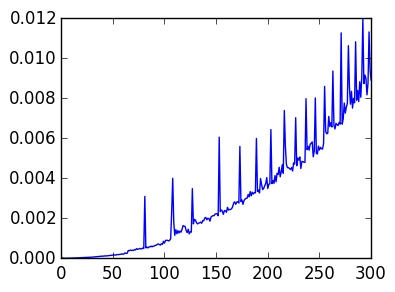A loglog plot scales both the x and y axis logarithmically, and is a useful tool for studying complexity, as the slope of $cn^\beta$ is dictated by $\beta$:

In :
r=1:10000

loglog(r,r)
loglog(r,2r)
loglog(r,r.^2)
loglog(r,2.5r.^2)
loglog(r,r.^3)
loglog(r,3r.^3);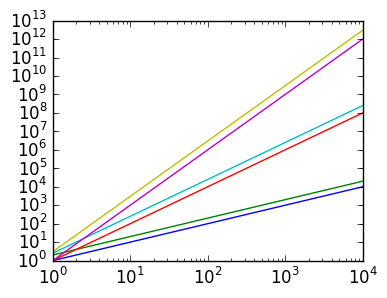It is also convenient to use a logarithmic choice of sample points, given by logspace(a,b,n), which returns n points between $10^a$ and $10^b$:

In :
r=logspace(0,4,10)  # 10 points sampled logarithmically between 1 and 10^4

loglog(r,r)
loglog(r,2r)
loglog(r,r.^2)
loglog(r,2.5r.^2)
loglog(r,r.^3)
loglog(r,3r.^3);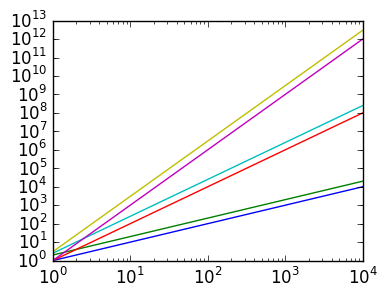Exercise 5(b) Construct a vector logtms of the time taken for QR on a $k \times k$ matrix, for k = floor(Int,j) for each j in logspace(0,3.2,10). Plot a loglog of the results.

In :
r=logspace(0,3.2,20)

N=length(r)
logtms=zeros(N)

for p=1:N
k=floor(Int,r[p])
A=rand(k,k)
logtms[p]=@elapsed (qr(A))
end

loglog(r,logtms);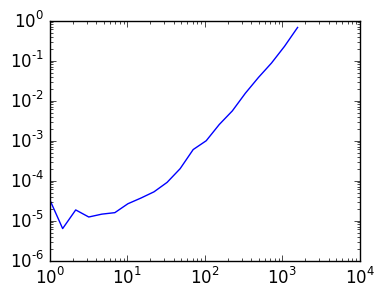Exercise 5(c) Can you guess the complexity of qr? That is, it has complexity of $O(n^\beta)$ for what choice of $\beta$? Verify your guess by comparing a loglog plot of $0.00000001n^\beta$ to the plot of logtms.

In :
k=logspace(0,4,10)

loglog(r,logtms)
loglog(r,0.00000001r.^3)
loglog(r,0.00000001r.^2);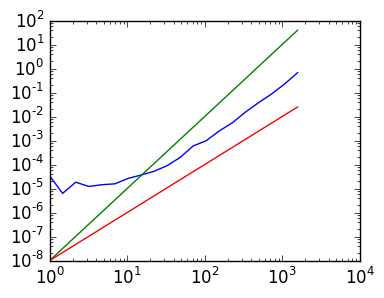Exercise 5(d) Repeat 5(c) for house_qr, for logspace(1,2.5,10) (for speed reasons).

In :
r=logspace(1,2.5,10)

N=length(r)
logtms=zeros(N)

for p=1:N
k=floor(Int,r[p])
A=rand(k,k)
logtms[p]=@elapsed (house_qr(A))
end

loglog(r,logtms)
loglog(r,0.00000001r.^3)
loglog(r,0.00000001r.^4);